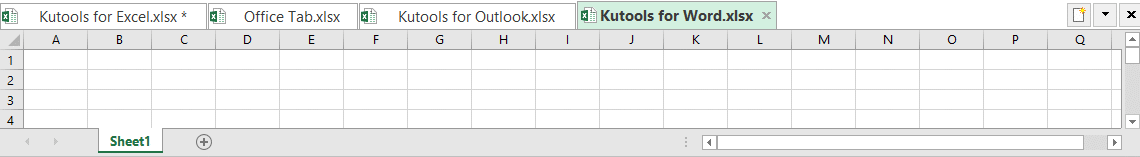Note: The other languages of the website are Google-translated. Back to English

## VLOOKUP function with some basic and advanced examples in Excel

In Excel, the VLOOKUP function is a powerful function for most of Excel users, which is used to look for a value in the leftmost of the data range, and return a matching value in the same row from a column you specified as below screenshot shown. This tutorial is talking about how to use the VLOOKUP function with some basic and advanced examples in Excel.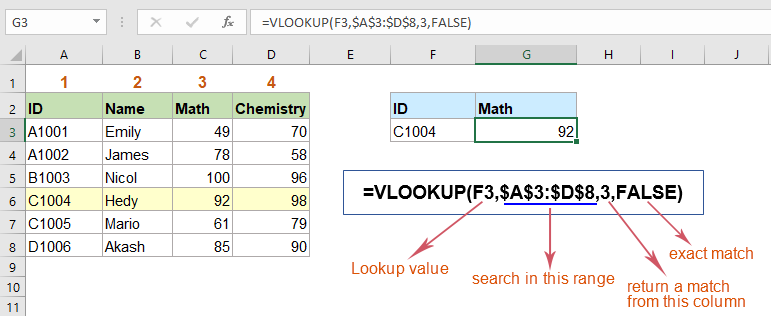### Introduction of VLOOKUP function – Syntax and Arguments

The syntax of VLOOKUP function:

=VLOOKUP (lookup_value, table_array, col_index_num, [range_lookup])

Arguments:

Lookup_value: The value that you want to search. It must be in the first column of the table_array range.

Table_array: The data range or table where the lookup value column and the result value column locating.

Col_index_num: The number of column from which the matched value will be returned. It starts with 1 from the leftmost column in the table array.

Range_lookup: A logical value that determines whether this VLOOKUP function will return an exact match or an approximate match.

• Approximate match1 / TRUE: If an exact match is not found, the formula searches for the closest match - the largest value that is smaller than the lookup value. In this case, you should sort the lookup column in ascending order.
=VLOOKUP(lookup_value, table_array, col_index, TRUE)
=VLOOKUP(lookup_value, table_array, col_index, 1)
• Exact match0 / FALSE: This is used to search for a value exactly equal to the lookup value. If an exact match is not found, the error value #N/A will be returned.
=VLOOKUP(lookup_value, table_array, col_index, FALSE)
=VLOOKUP(lookup_value, table_array, col_index, 0)

Notes:

• 1. The Vlookup function only looks for value from left to right.
• 2. If there are multiple matching values based on the look up value, only the first matched will be returned by using the Vlookup function.
• 3. It will return the #N/A error value if the look up value can’t be found.

### Basic VLOOKUP examples

#### 1. Do an exact match Vlookup and approximate match Vlookup

###### Do an exact match Vlookup in Excel

Normally, if you are looking for an exact match with the Vlookup function, you just need to use FALSE in the last argument.

For example, to get the corresponding Math scores based on the specific ID numbers, please do as this: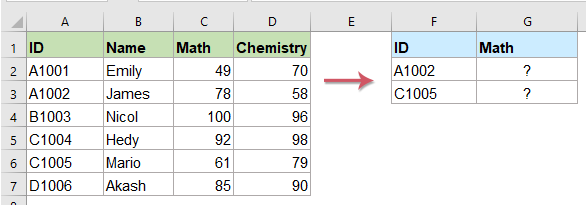1. Apply the below formula into a blank cell where you want to get the result:

=VLOOKUP(F2,\$A\$2:\$D\$7,3,FALSE)

2. And then, drag the fill handle down to the cells you want to fill this formula, and you will get the results as you need. See screenshot: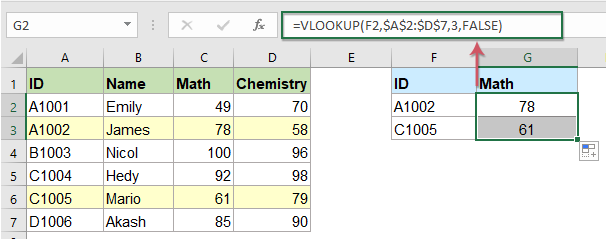Notes:

• 1. In the above formula, F2 is the value that you want to return its matching value, A2:D7 is the table array, the number 3 is the column number that your matched value is returned from and the FALSE refers to the exact match.
• 2. If your criteria value is not found in the data range, an error value #N/A will be displayed.

###### Do an approximate match Vlookup in Excel

The approximate match is useful for searching values between data ranges. If the exact match is not found, the approximate Vlookup will return the largest value that is smaller than the lookup value.

For example, if you have the following range data, the specified orders are not in the Orders column, how to get its closest Discount in column B?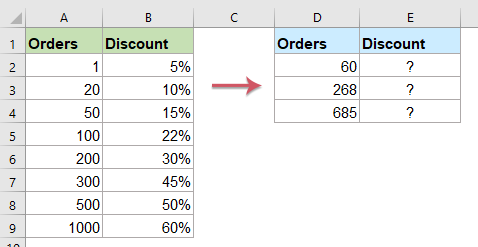1. Enter the following formula into a cell where you want to put the result:

=VLOOKUP(D2,\$A\$2:\$B\$9,2,TRUE)

2. Then, drag the fill handle down to the cells to apply this formula, and you will get the approximate matches based on the given values, see screenshot: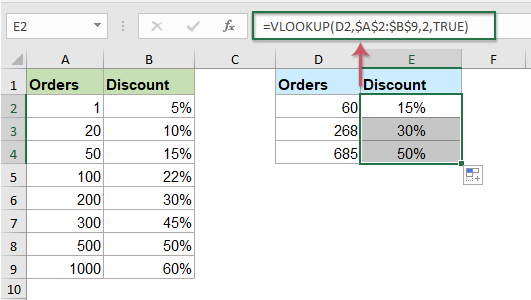Notes:

• 1. In the above formula, D2 is the value that you want to return its relative information, A2:B9 is the data range, the number 2 indicates the column number that your matched value is returned and the TRUE refers to the approximate match.
• 2. The approximate match will return the largest value that is smaller than your specific lookup value.
• 3. To use the Vlookup function to get an approximate match value, you must sort the leftmost column of the data range in ascending order, otherwise it will return a wrong result.

#### 2. Do a case sensitive Vlookup in Excel

By default, the Vlookup function performs a case insensitive lookup which means it treats lowercase and uppercase characters as identical. Sometime, you may need to do a case sensitive lookup in Excel, the Index, Match and Exact functions or Lookup and Exact functions can do you a favor.

For example, I have the following data range which ID column contains text string with upper case or lower case, now, I want to return the corresponding Math score of the given ID number.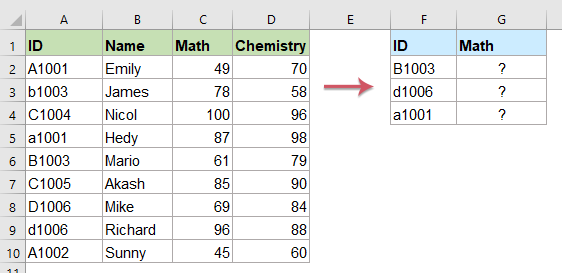Formula 1: Using EXACT, INDEX, MATCH functions

1. Please enter or copy the below array formula into a blank cell where you want to get the result:

=INDEX(\$C\$2:\$C\$10,MATCH(TRUE,EXACT(F2,\$A\$2:\$A\$10),0))

2. Then, press Ctrl + Shift + Enter keys simultaneously to get the first result, and then select the formula cell, drag the fill handle down to the cells you want to fill this formula, then you will get the correct results you need. See screenshot: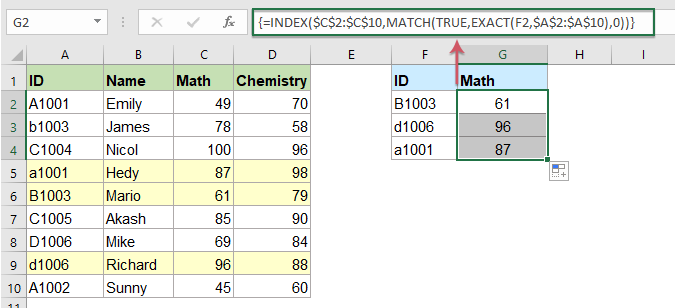Notes:

• 1. In the above formula, A2:A10 is the column which contains the specific values you want to look up in, F2 is the lookup value, C2:C10 is the column where the result will be returned from.
• 2. If multiple matches found, this formula will always return the first match.

Formula 2: Using Lookup and Exact functions

1. Please apply the below formula into a blank cell where you want to get the result:

=LOOKUP(2,1/EXACT(F2,\$A\$2:\$A\$10),\$C\$2:\$C\$10)

2. Then, drag the fill handle down to the cells that you want to copy this formula, and you will get the matched values with case sensitive as below screenshot shown: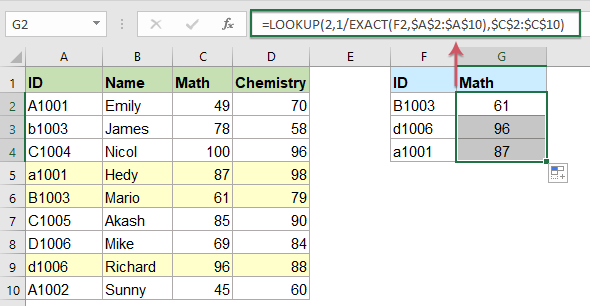Notes:

• 1. In the above formula, A2:A10 is the column which contains the specific values you want to look up in, F2 is the lookup value, C2:C10 is the column where the result will be returned from.
• 2. If multiple matches found, this formula will always return the last match.

#### 3. Vlookup values from right to left in Excel

The Vlookup function always looks up a value in the leftmost column of a data range and returns the corresponding value from a column to the right. If you want to do a reverse Vlookup which means to lookup a specific value in the right and return its corresponding value in left column as below screenshot shown: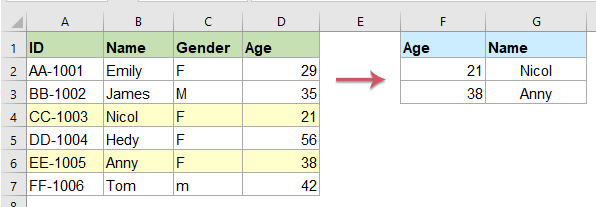#### 4. Vlookup the second, nth or last matching value in Excel

Normally, if there are multiple matching values are found when using the Vlookup function, only the first matched record will be returned. In this section, I will talk about how to get the second, nth or last matching value with the Vlookup function.

###### Vlookup and return the second or nth matching value

Suppose you have a list of names in column A, the training course they purchased in column B, and now, you are looking to find the 2nd or nth training course bought by the given customer. See screenshot: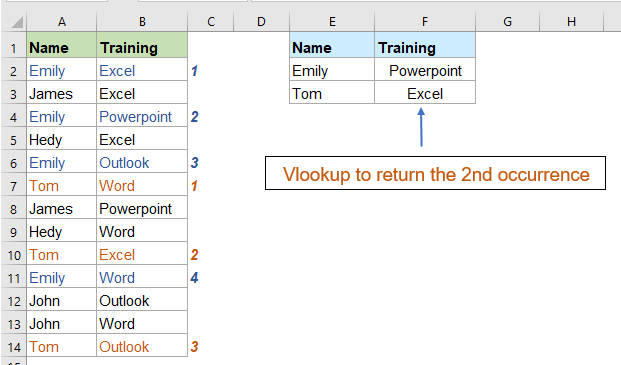1. To get the second or nth matching value based on the given criteria, please apply the following array formula into a blank cell:

=INDEX(\$B\$2:\$B\$14,SMALL(IF(E2=\$A\$2:\$A\$14,ROW(\$A\$2:\$A\$14)-ROW(\$A\$2)+1),2))

2. Then press Ctrl + Shift + Enter keys together to get the first result, and then select the formula cell, drag the fill handle down to the cells you want to fill this formula, and all the second matched values based on the given names have been displayed at once, see screenshot: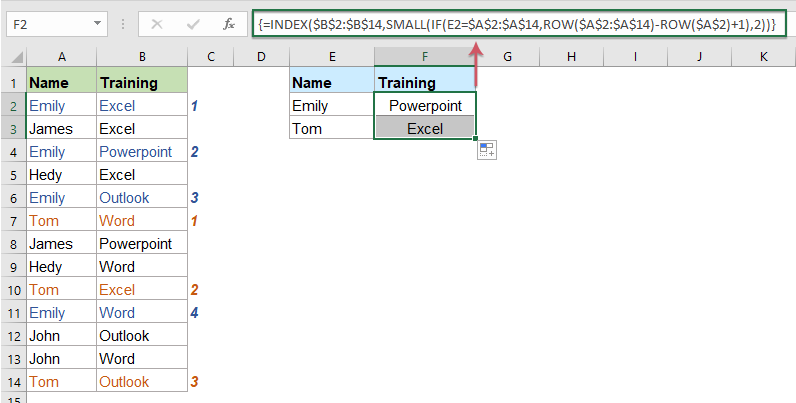Note:

• In this formula, A2:A14 is the range with all the values for lookup, B2:B14 is the range of the matching values you want to return from, E2 is the lookup value, and the last number 2 indicates the second matched value you want to get, if you want to want to return the third matching value, you just need to change it to 3 as you need.

###### Vlookup and return the last matching value

If you want to vlookup and return the last matching value as below screenshot shown, this Vlookup And Return The Last Matching Value tutorial may help you to get last matching value in details.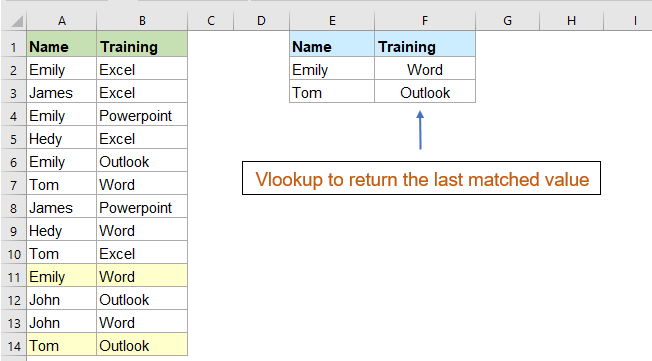#### 5. Vlookup matching values between two given values or dates

Sometimes, you may want to lookup values between two values or dates and return the corresponding results as below screenshot shown, in this case, you can use the LOOKUP function and a sorted table.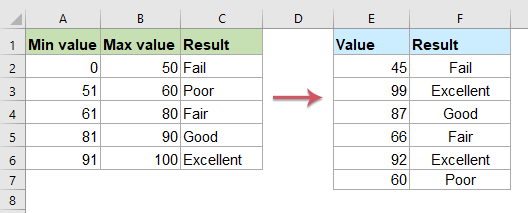###### Vlookup matching values between two given values or dates with formula

1. First, your original table should be a sorted data range. And then, copy or enter the following formula into a blank cell:

=LOOKUP(2,1/(\$A\$2:\$A\$6<=E2)/(\$B\$2:\$B\$6>=E2),\$C\$2:\$C\$6)

2. Then, drag the fill handle to fill this formula to other cells you need, and now, you will get all matched records based on the given value, see screenshot: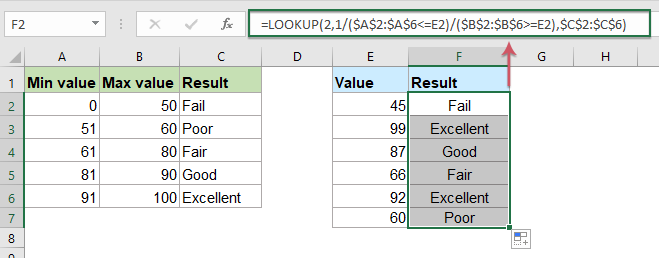Notes:

• 1. In the above formula, A2:A6 is the range of smaller values and B2:B6 is the range of larger numbers in your data range, the E2 is the given value which you want to get its corresponding value, C2:C6 is the column data which you want to extract from.
• 2. This formula also can be used for extracting matched values between two dates as below screenshot shown: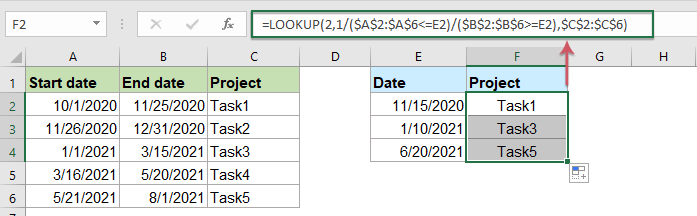###### Vlookup matching values between two given values or dates with a useful feature

If you are painful with the above formula, here, I will introduce an easy tool – Kutools for Excel, with its LOOKUP between Two Values feature, you can return the corresponding item based on the specific value or date between two values or dates without remembering any formula.   Click to download Kutools for Excel now!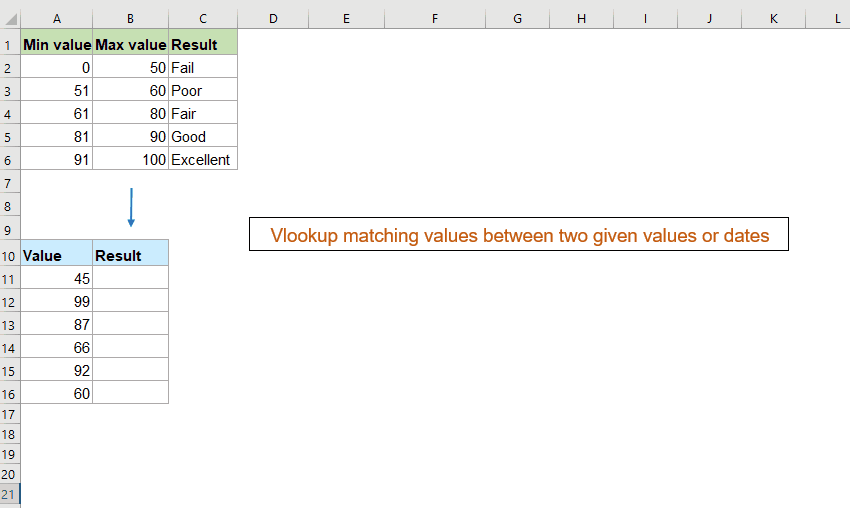#### 6. Using wildcards for partial matches in Vlookup function

In Excel, the wildcards can be used within the Vlookup function, which makes to perform a partial match on a lookup value. For instance, you can use Vlookup to return matched value from a table based on part of a lookup value.

Supposing, I have a range of data as below screenshot shown, now, I want to extract the score based on the first name (not full name). How could solve this task in Excel?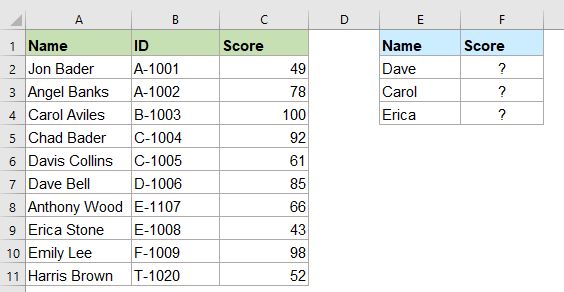1. The normal Vlookup function does not work correctly, you need joins the text or cell reference with a wildcard, please copy or enter the following formula into a blank cell:

=VLOOKUP(E2&"*", \$A\$2:\$C\$11, 3, FALSE)

2. Then, drag the fill handle to fill this formula to other cells you need, and all the matched scores have been returned as below screenshot shown: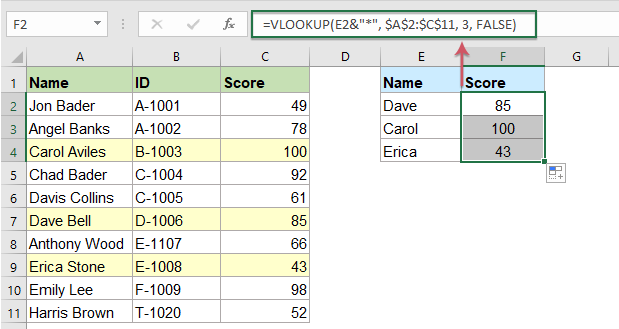Notes:

• 1. In the above formula, E2&”*” is the lookup value, the value in E2 and the * wildcard (“*” indicates any one character or any characters), A2:C11 is the lookup range, the number 3 the column that contains the value to return.
• 2. Vlookup when using wildcards, you must set exact match mode with FALSE or 0 for the last argument in Vlookup function.

Tips:

1. Find and return the matching values ending with a specific value, please apply this formula: =VLOOKUP("*"&E2, \$A\$2:\$C\$11, 3, FALSE)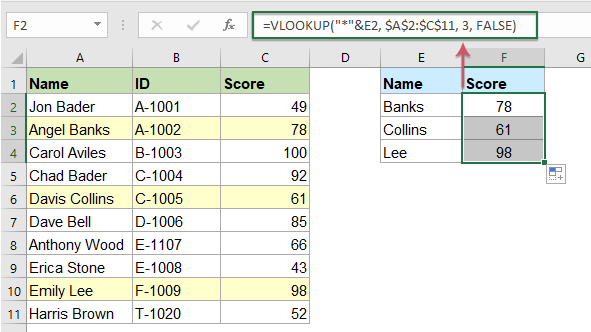2. To lookup and return the matched value based on part of the text string, whether the specified text is in front, behind or in middle of the text string, you just need to join two * characters around the cell reference or text. Please do with this formula: =VLOOKUP("*"&D2&"*", \$A\$2:\$B\$11, 2, FALSE)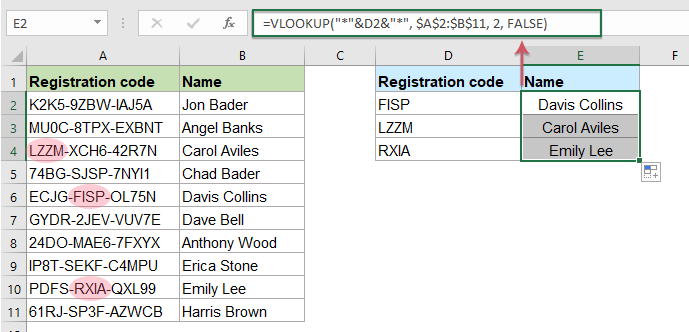#### 7. Vlookup values from another worksheet

Usually, you may have to work with more than one worksheet, the Vlookup function can be used to lookup data from another sheet as the same as on one worksheet.

For example, you have two worksheets as below screenshot shown, to lookup and return the corresponding data from the worksheet you specified, please do with the following steps: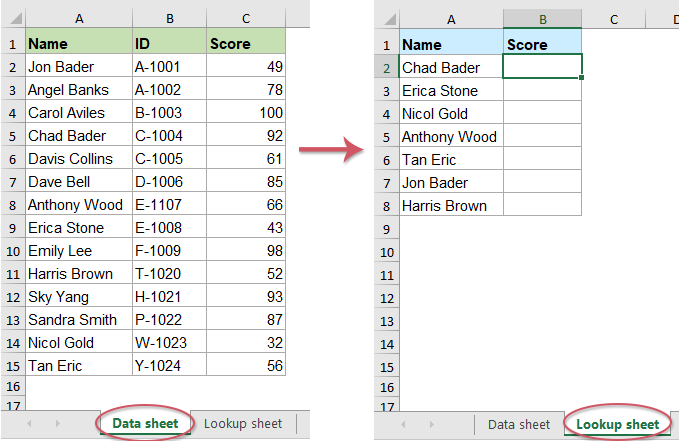1. Please enter or copy the below formula into a blank cell where you want to get the matched items:

=VLOOKUP(A2,'Data sheet'!\$A\$2:\$C\$15,3,0)

2. Then, drag the fill handle down to the cells that you want to apply this formula, and you will get the corresponding results as you need, see screenshot: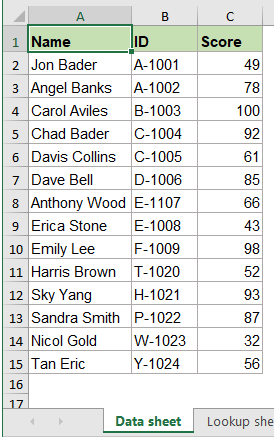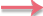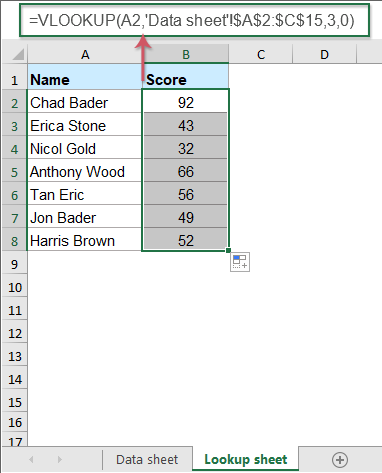Note: In the above formula:

• A2 represents the lookup value;
• Data sheet is the name of the worksheet that you want to lookup data from, (If the sheet name contains space or punctuation characters, you should enclose single quotes around the sheet name, otherwise, you can directly use the sheet name like =VLOOKUP(A2,Datasheet!\$A\$2:\$C\$15,3,0) );
• A2:C15 is the range of data in Data Sheet where we are searching data for;
• the number 3 is the column number which contains matched data you want to return from.

#### 8. Vlookup values from another workbook

This section will talk about lookup and return the matching values from a different workbook by using the Vlookup function.

For example, the first workbook contains the product and cost lists, now, you want to extract the corresponding cost in the second workbook based on the product item as below screenshot shown.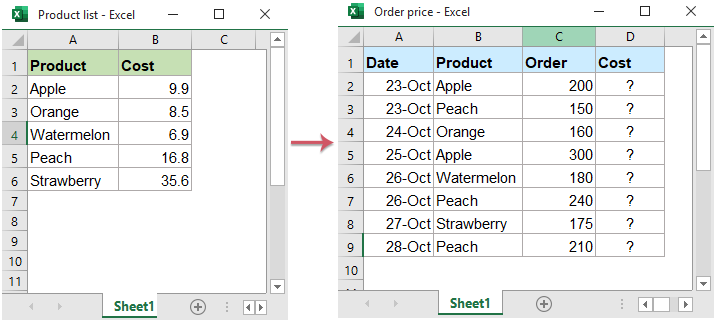1. To retrieve the relative cost from another workbook, first, open both the workbooks you want to use, then apply the following formula into a cell where you want to put the result:

=VLOOKUP(B2,'[Product list.xlsx]Sheet1'!\$A\$2:\$B\$6,2,0)

2. Then, drag and copy this formula to other cells you need, see screenshot: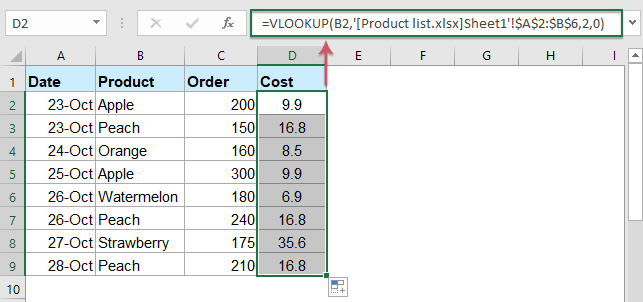Notes:

• 1. In the above formula:
B2 represents the lookup value;
[Product list.xlsx]Sheet1 is the name of the workbook and worksheet that you want to lookup data from, (The reference to workbook is enclosed in square brackets, and the entire workbook + sheet is enclosed in single quotes);
A2:B6 is the range of data in the worksheet of another workbook where we are searching data for;
the number 2 is the column number which contains matched data you want to return from.
• 2. If the lookup workbook is closed, the full file path for the lookup workbook will be shown in the formula as following screenshot shown: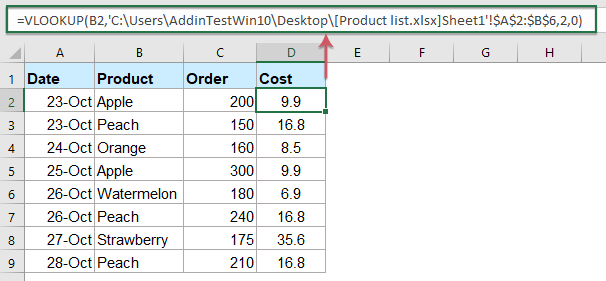#### 9. Vlookup and return blank or specific text instead of 0 or #N/A error value

Normally, when you apply the vlookup function to return the corresponding value, if your matching cell is blank, it will return 0, and if your matching value is not found, you will get an error #N/A value as below screenshot shown. Instead of displaying the 0 or #N/A value with blank cell or other value you like, this Vlookup To Return Blank Or Specific Value Instead Of 0 Or N/A tutorial may do you a favor step by step.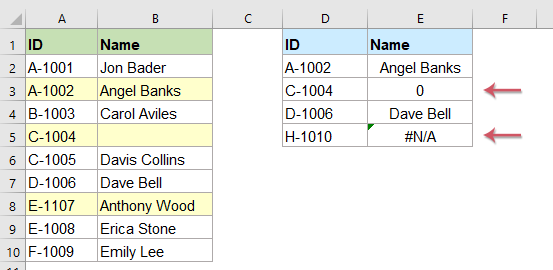#### 1. Two-way lookup with Vlookup function (Vlookup in row and column)

Sometimes, you may need do a 2-dimensional lookup, which means to Vlookup in both row and column at the same time. Let say, if you have the following data range, and now, you may need to get the value for a particular product in a specified quarter. This section will introduce some formula for dealing with this job in Excel.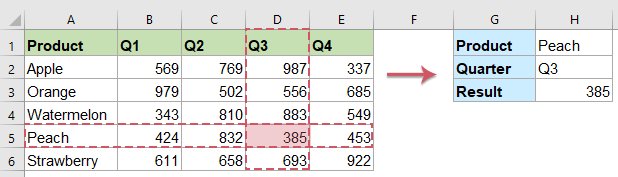Formula 1: Using VLOOKUP and MATCH functions

In Excel, you can use a combination of VLOOKUP and MATCH functions to do a two-way lookup, please apply the following formula into a blank cell, and then press Enter key to get the result.

=VLOOKUP(H1, \$A\$2:\$E\$6, MATCH(H2, \$A\$1:\$E\$1, 0), FALSE)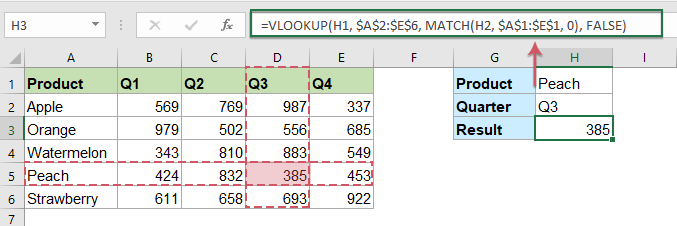Note: In the above formula:

• H1: the lookup value in the column that you want to get the corresponding value based on;
• A2:E6: the data range including row headers;
• H2: the lookup value in the row that you want to get the corresponding value based on;
• A1:E1: the cells of column headers.

Formula 2: Using INDEX and MATCH functions

Here is another formula also can help you to perform a 2-dimensional lookup, please apply the below formula, and then press Enter key to get the result you need.

=INDEX(\$B\$2:\$E\$6, MATCH(H1, \$A\$2:\$A\$6, 0), MATCH(H2, \$B\$1:\$E\$1, 0))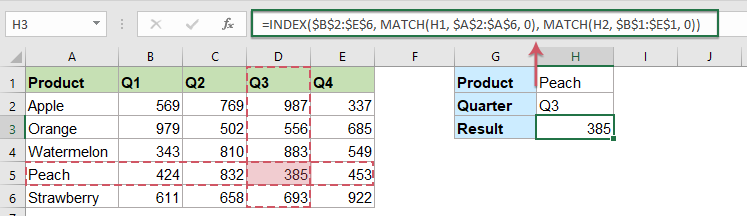Note: In the above formula:

• B2:E6: the data range to return the matched item from;
• H1: the lookup value in the column that you want to get the corresponding value based on;
• A2:A6: the row headers contain the product you want to look for.
• H2: the lookup value in the row that you want to get the corresponding value based on;
• B1:E1: the column headers contain the quarter you want to look for.

#### 2. Vlookup matching value based on two or more criteria

It is easy for you to look up the matching value based on one criterion, but if you have two or more criteria, what can you do? The LOOKUP or MATCH and INDEX functions in Excel can help you to solve this job quickly and easily.

For example, I have the below data table, to return the matched price based on the specific product and size, the following formulas may help you.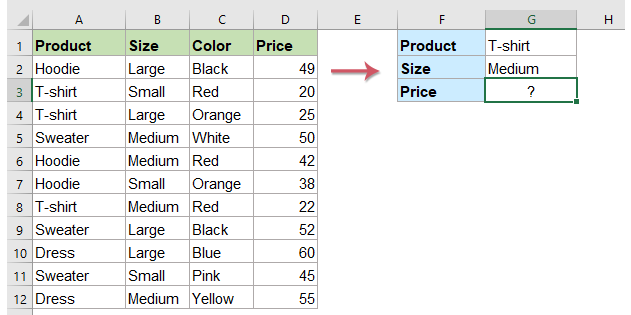Formula 1: Using LOOKUP function

Please apply the below formula into a cell where you want to get the result, and then press Enter key, see screenshot:

=LOOKUP(2,1/(\$A\$2:\$A\$12=G1)/(\$B\$2:\$B\$12=G2),(\$D\$2:\$D\$12))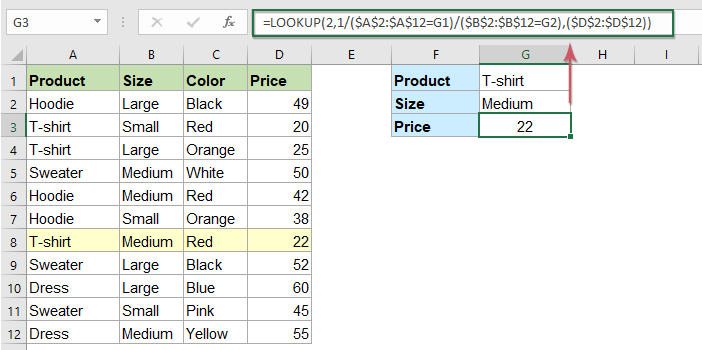Notes:

• 1. In the above formula:
A2:A12=G1: means to search the criteria of G1 in range A2:A12;
B2:B12=G2: means to search the criteria of G2 in range B2:B12;
D2:D12: the range which you want to return the corresponding value.
• 2. If you have more than two criteria, you just need to join the other criteria into the formula, such as: =LOOKUP(2,1/(\$A\$2:\$A\$12=G1)/(\$B\$2:\$B\$12=G2)/(\$C\$2:\$C\$12=G3),(\$D\$2:\$D\$12))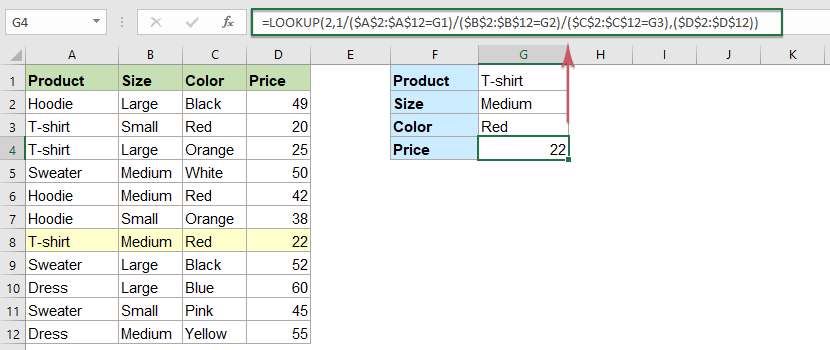Formula 2: Using INDEXT And MATCH functions

The combination of Index and Match function also can be used to return the matched value based on multiple criteria. Please copy or enter the following formula:

=INDEX(\$D\$2:\$D\$12,MATCH(1,(\$A\$2:\$A\$12=G1)*(\$B\$2:\$B\$12=G2),0))

Then, press Ctrl + Shift + Enter keys together to get the relative value as you need. See screenshot: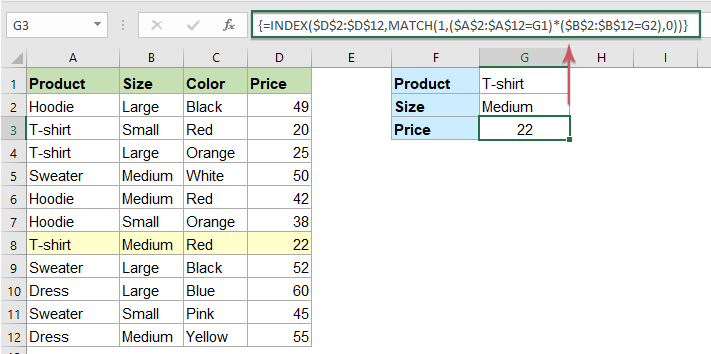Notes:

• 1. In the above formula:
A2:A12=G1: means to search the criteria of G1 in range A2:A12;
B2:B12=G2: means to search the criteria of G2 in range B2:B12;
D2:D12: the range which you want to return the corresponding value.
• 2. If you have more than two criteria, you just need to join the new criteria into the formula, such as: =INDEX(\$D\$2:\$D\$12,MATCH(1,(\$A\$2:\$A\$12=G1)*(\$B\$2:\$B\$12=G2)*(\$C\$2:\$C\$12=G3),0))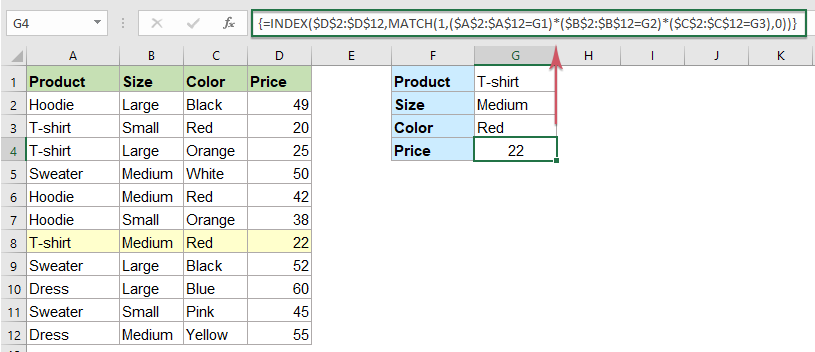#### 3. Vlookup to return multiple matching values with one or more conditions

In Excel, the Vlookup function searches for a value and only return the first matching value if there are multiple corresponding values found. Sometimes, you may want to return all the corresponding values in a row, in a column or in a single cell. This section will talk about how to return the multiple matching values with one or more conditions in a workbook.

###### Vlookup all matching values based on one or more conditions horizontally

Vlookup all matching values based on one condition horizontally:

To Vlookup and return all matching values based on one specific value horizontally, the generic formula is:

=IFERROR(INDEX(return_range, SMALL(IF(lookup_value = lookup_range, ROW(return_range) - m, ""), COLUMN() - n)), "")
Note: m is the row number of the first cell in the return range minus 1.
n is the column number of the first formula cell minus 1.

1. Please apply the below formula into a blank cell, then press Ctrl + Shift + Enter keys together to get the first matched value, see screenshot:

=IFERROR(INDEX(\$C\$2:\$C\$20, SMALL(IF(\$F1=\$A\$2:\$A\$20, ROW(\$C\$2:\$C\$20)-1,""), COLUMN()-5)),"")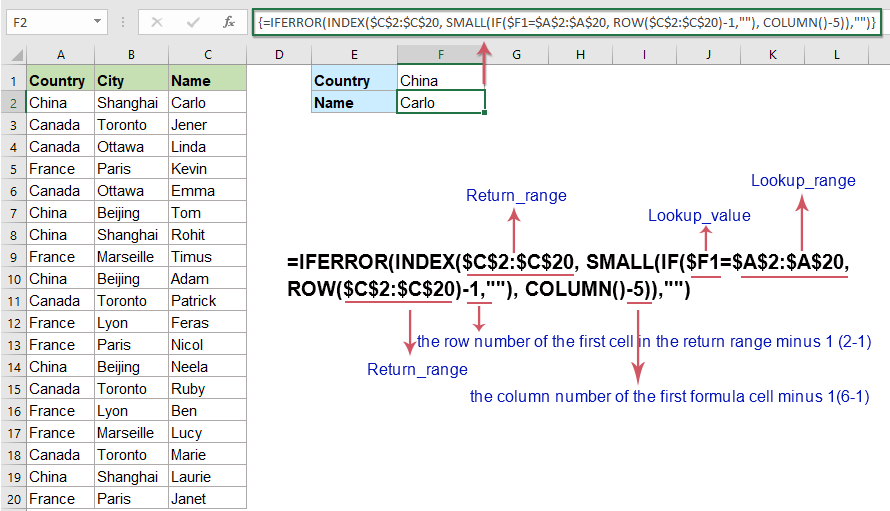2. And then, select the first formula cell, and drag the fill handle to right cells until blank cell is displayed, and all corresponding items have been extracted, see screenshot: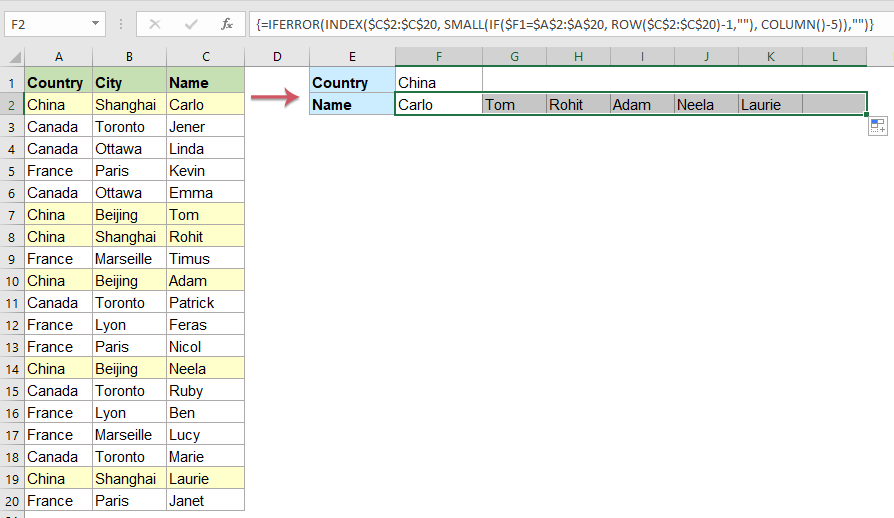Tips:

If there are duplicate matched values in the returned list, to ignore the duplicates, please use this formulas, then press Enter to get the first result: =IFERROR(INDEX(\$C\$2:\$C\$20,MATCH(\$F1,\$A\$2:\$A\$20,0)),"")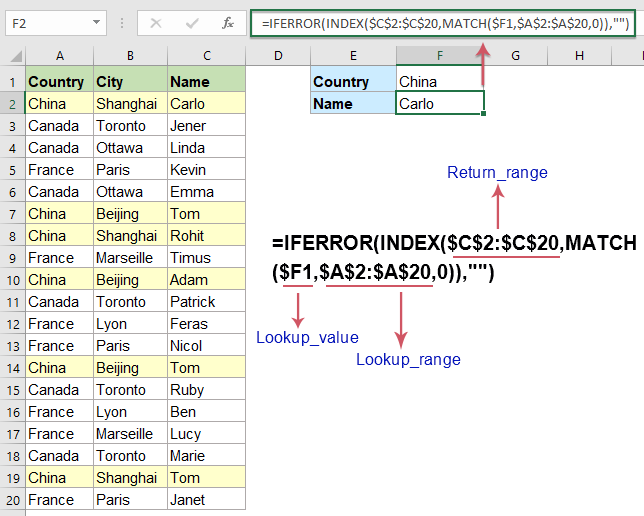Go on entering this formula: =IFERROR(INDEX(\$C\$2:\$C\$20,MATCH(1,(\$F1=\$A\$2:\$A\$20)*ISNA(MATCH(\$C\$2:\$C\$20,\$F2:F2,0)),0)),"") into a cell beside the first result, and then press Ctrl + Shift + Enter keys together to get the second result, then drag this formula to right cells to get all other matched values until blank cell displays, see screenshot: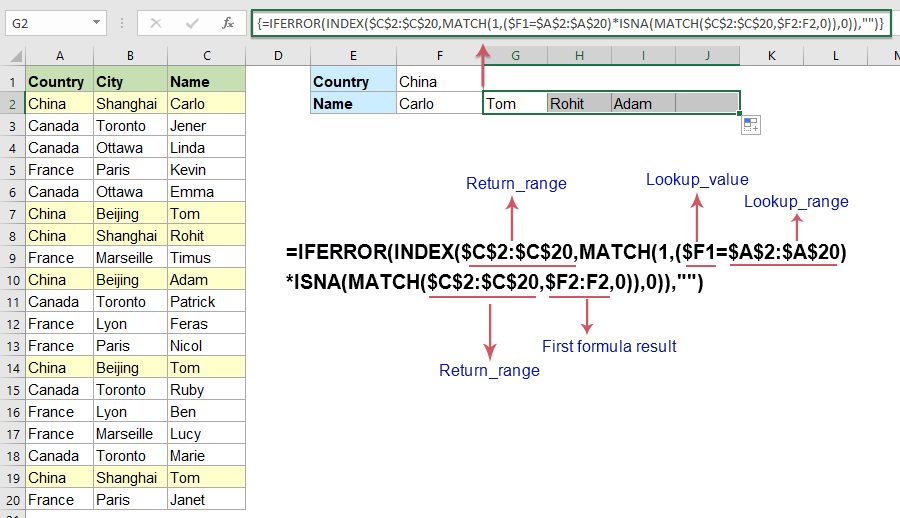Vlookup all matching values based on two or more conditions horizontally:

To Vlookup and return all matching values based on more specific values horizontally, the generic formula is:

=IFERROR(INDEX(return_range, SMALL(IF(1 = ((--(lookup_value1=lookup_range1)) * (--(lookup_value2 = lookup_range2))), ROW(return_range) - m, ""), COLUMN() - n)),"")
Note: m is the row number of the first cell in the return range minus 1.
n is the column number of the first formula cell minus 1.

1. Apply the following formula into a blank cell where you want to output the result:

=IFERROR(INDEX(\$C\$2:\$C\$20, SMALL(IF(1=((--(\$F1=\$A\$2:\$A\$20)) * (--(\$F2=\$B\$2:\$B\$20))), ROW(\$C\$2:\$C\$20)-1,""), COLUMN()-5)),"")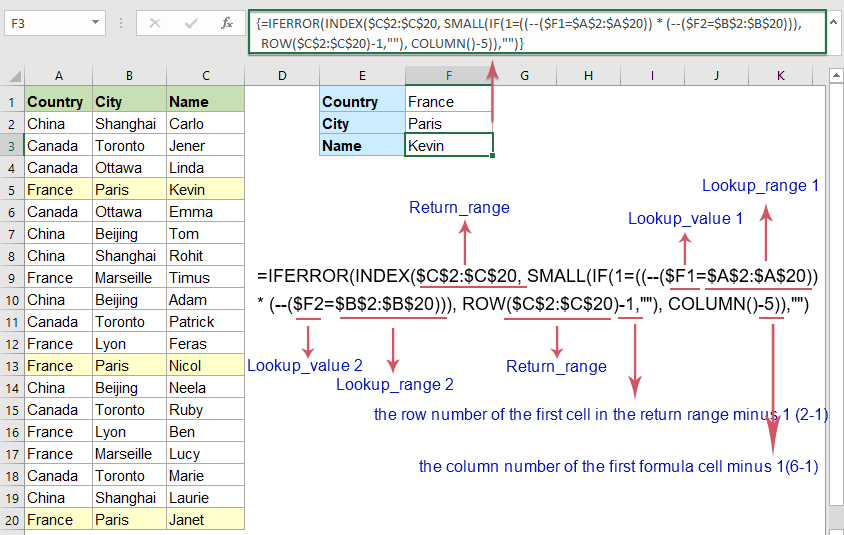2. Then, select the formula cell and drag the fill handle to right cells until blank cell displays, and all matched values based on the specific criteria have been returned, see screenshot: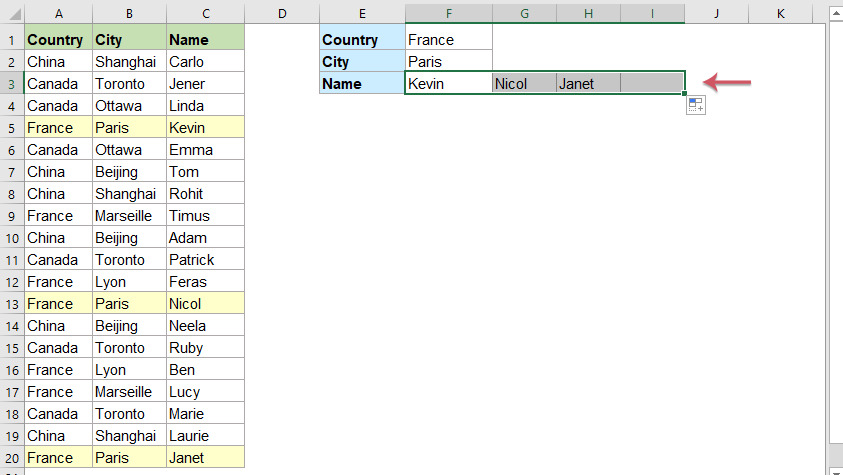Note: For more criteria, you just need to join the lookup_value and lookup_range into the formula, such as: =IFERROR(INDEX(return_range, SMALL(IF(1 = ((--(lookup_value1=lookup_range1)) * (--(lookup_value2 = lookup_range2) * (--(lookup_value3 =lookup_range3)))), ROW(return_range) - m, ""), COLUMN() - n)),"").

###### Vlookup all matching values based on one or more conditions vertically

Vlookup all matching values based on one condition vertically:

To Vlookup and return all matching values based on one specific value vertically, the generic formula is:

=IFERROR(INDEX(return_range, SMALL(IF(lookup_value = lookup_range, ROW(return_range )- m ,""), ROW() - n )),"")
Note: m is the row number of the first cell in the return range minus 1.
n is the row number of the first formula cell minus 1.

1. Copy or type the following formula into a cell where you want to get the result, and then press Ctrl + Shift + Enter keys together to get the first matched value, see screenshot:

=IFERROR(INDEX(\$C\$2:\$C\$20, SMALL(IF(E\$2=\$A\$2:\$A\$20, ROW(\$C\$2:\$C\$20)-1,""), ROW()-1)),"")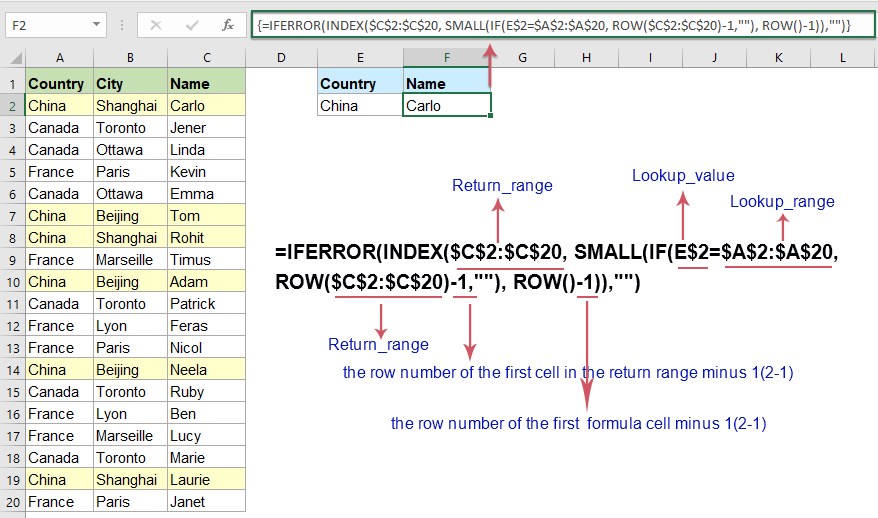2. Then, select the first formula cell, and drag the fill handle down to other cells until blank cell is displayed, and all corresponding items have been listed in a column, see screenshot: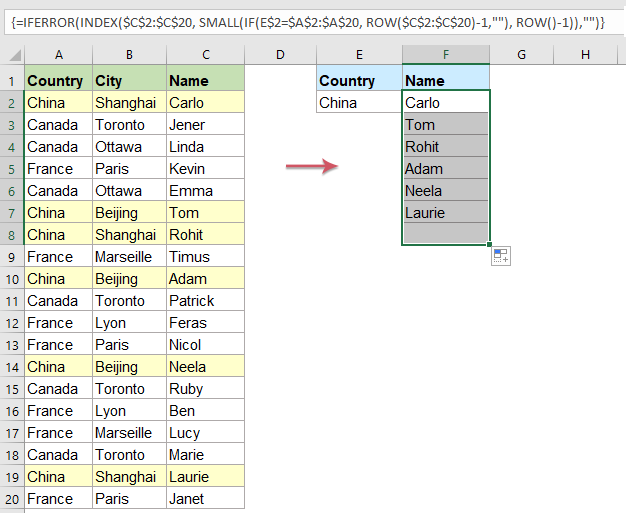Tips:

To ignore the duplicates in the returned matching values, please use this formulas: =IFERROR(INDEX(\$C\$2:\$C\$20,MATCH(0,COUNTIF(\$F\$1:F1,\$C\$2:\$C\$20)+(\$A\$2:\$A\$20<>\$E\$2),0)),"")

Then press Ctrl + Shift + Enter keys together to get the first matched value, and then drag this formula cell down to other cells until blank cell displays, and you will get the result as you need: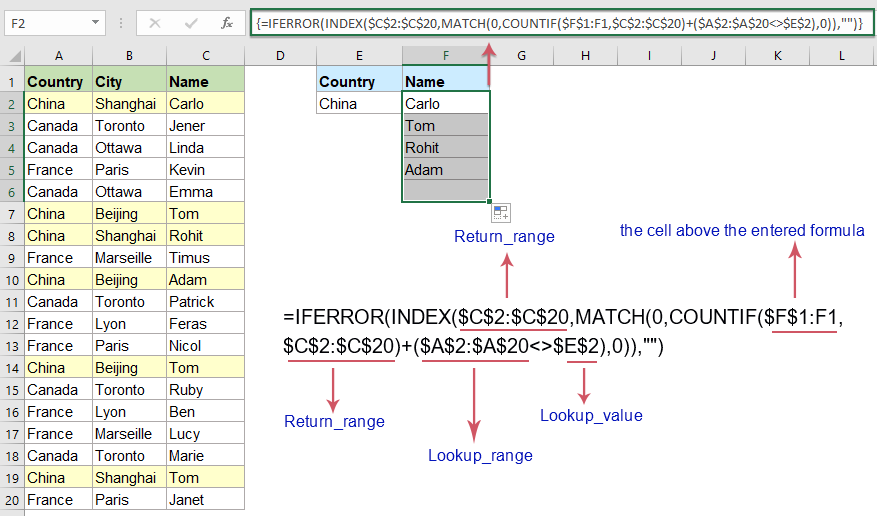Vlookup all matching values based on two or more conditions vertically:

To Vlookup and return all matching values based on more specific values vertically, the generic formula is:

=IFERROR(INDEX(return_range, SMALL(IF(1=((--(lookup_value1=lookup_range1)) * ( --(lookup_value2=lookup_range2))), ROW(return_range)-m,""), ROW()-n)),"")
Note: m is the row number of the first cell in the return range minus 1.
n is the row number of the first formula cell minus 1.

1. Copy the below formula into a blank cell, and then press Ctrl + Shift + Enter keys together to get the first matched item.

=IFERROR(INDEX(\$C\$2:\$C\$20, SMALL(IF(1=((--(\$E\$2=\$A\$2:\$A\$20)) * (--(\$F\$2=\$B\$2:\$B\$20))), ROW(\$C\$2:\$C\$20)-1,""), ROW()-1)),"")2. Then drag the formula cell down to other cells until blank cell is displayed, see screenshot: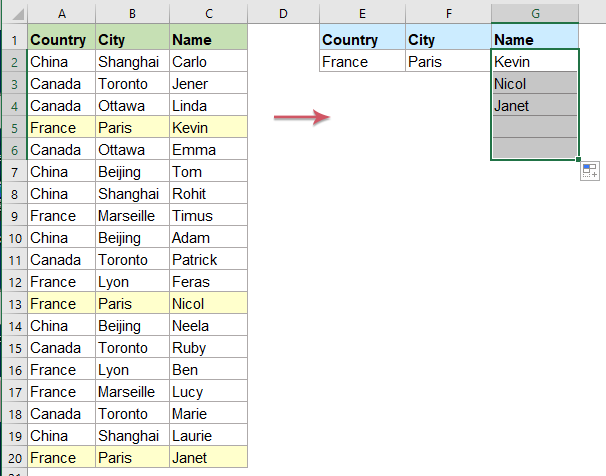Note: For more criteria, you just need to join the lookup_value and lookup_range into the formula, such as: =IFERROR(INDEX(return_range, SMALL(IF(1 = ((--(lookup_value1=lookup_range1)) * (--(lookup_value2 = lookup_range2) * (--(lookup_value3 =lookup_range3)))), ROW(return_range) - m, ""), ROW() - n)),"").

###### Vlookup all matching values based on two or more conditions into single cell

If you want to Vlookup and return multiple matched values into a single cell with specified separator, the new function of TEXTJOIN can help you to solve this job quickly and easily.

Vlookup all matching values based on one condition into single cell:

Please apply the below simple formula into a blank cell, and then press Ctrl + Shift + Enter keys together to get the result:

=TEXTJOIN(",",TRUE,IF(\$A\$2:\$A\$20=F1,\$C\$2:\$C\$20,""))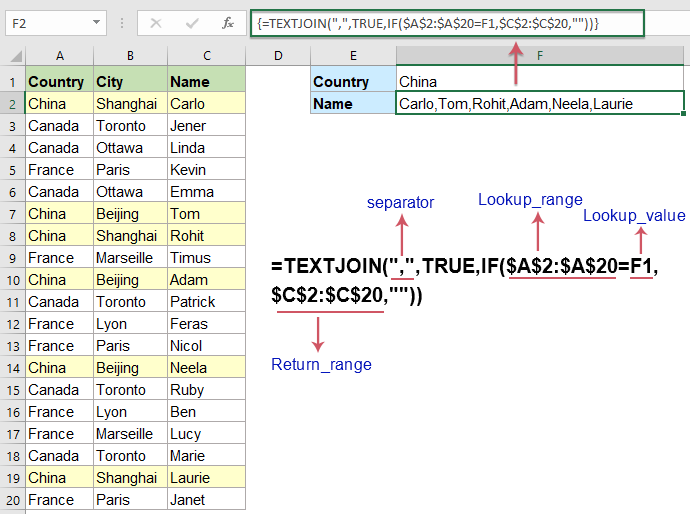Tips:

To ignore the duplicates in the returned matching values, please use this formulas: =TEXTJOIN(",", TRUE, IF(IFERROR(MATCH(\$C\$2:\$C\$20, IF(F1=\$A\$2:\$A\$20, \$C\$2:\$C\$20, ""), 0),"")=MATCH(ROW(\$C\$2:\$C\$20), ROW(\$C\$2:\$C\$20)), \$C\$2:\$C\$20, ""))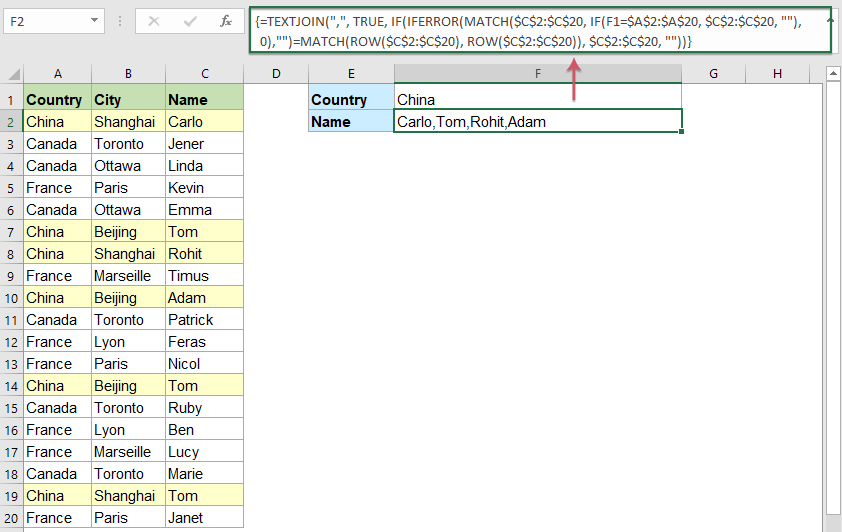Vlookup all matching values based on two or more conditions into single cell:

For dealing with multiple conditions when returning all matching values into a single cell, please apply the below formula, and then press Ctrl + Shift + Enter keys together to get the result:

=TEXTJOIN(",",TRUE,IF((\$A\$2:\$A\$20=F1)*(\$B\$2:\$B\$20=F2),\$C\$2:\$C\$20,""))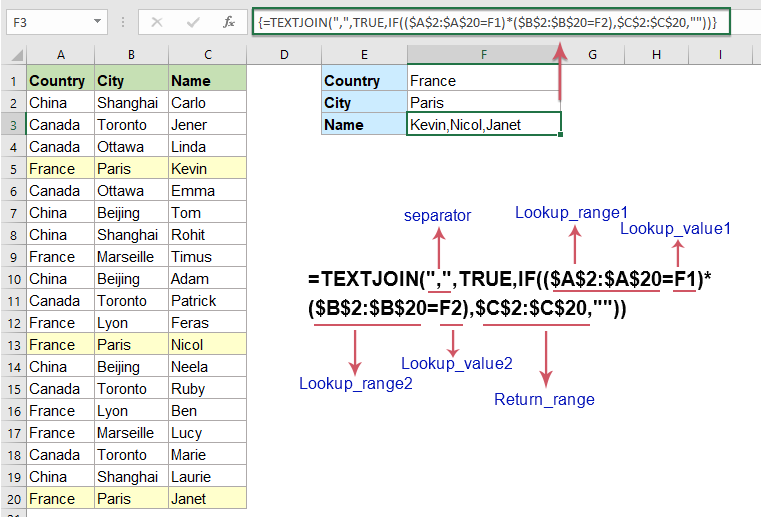Notes:

1. The TEXTJOIN function is only available in Excel 2019 and Office 365.

2. If you use the Excel 2016 and earlier versions, please use the User Defined Function of below articles:

#### 4. Vlookup to return entire or whole row of a matched cell

In this section, I will talk about how to retrieve the entire row of a matched value by using the Vlookup function.

1. Please copy or type the below formula into a blank cell where you want to output the result, and press Enter key to get the first value.

=VLOOKUP(\$F\$2,\$A\$1:\$D\$12,COLUMN(A1),FALSE)

2. Then, drag the formula cell to right side until the data of the entire row is displayed, see screenshot: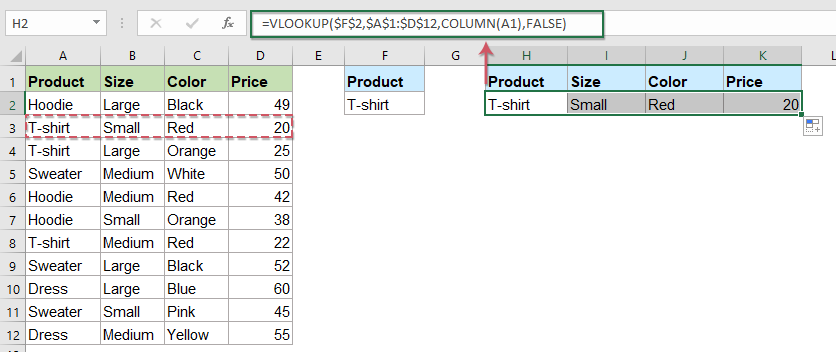Note: In the above formula, F2 is the lookup value you want to return the whole row based on, A1:D12 is the data range you want to use, A1 indicates the first column number within your data range.

Tips:

If multiple rows found based on the matched value, to return all of the corresponding rows, please apply this formula: =IFERROR(INDEX(A:A,SMALL(IF(ISNUMBER(SEARCH(\$F\$2,\$A\$2:\$A\$12)),ROW(\$A\$2:\$A\$12),""),ROW()-1)),""), and then press Ctrl + Shift + Enter keys together to get the first result, then drag the fill handle right to the cells, see screenshot: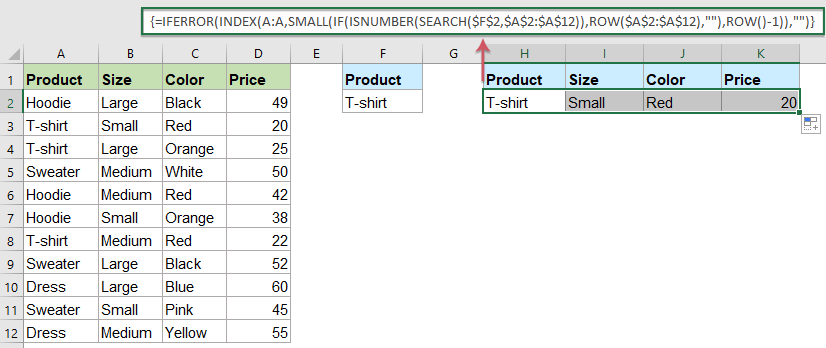And then drag the fill handle down across the cells to get all matching rows as below screenshot shown: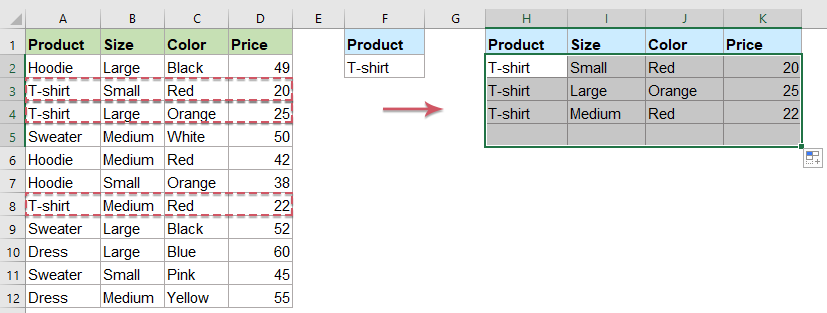#### 5. Do multiple Vlookup function (nested Vlookup) in Excel

Sometimes, you may want to look up values in multiple tables, if any of the tables contains the given lookup value as below screenshot shown, in this case, you can combine one or more Vlookup functions together with IFERROR function to perform multiple lookup.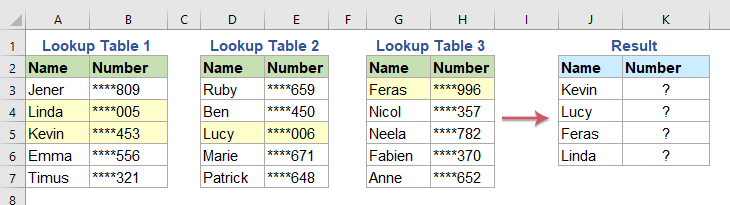The generic formula for nested Vlookup function is:

=IFERROR(VLOOKUP(lookup_value,table1,col,0),IFERROR(VLOOKUP(lookup_value,table2,col,0),VLOOKUP(lookup_value,table3,col,0)))

Note:

• lookup_value: the value you are looking for;
• Table1, Table2, Table3,...: the tables in which the lookup value and return value exist;
• col: the column number in the table from which you want to return the matching value.
• 0: This is used for an exact match.

1. Please apply the following formula into a blank cell where you want to put the result:

=IFERROR(VLOOKUP(J3,\$A\$3:\$B\$7,2,0),IFERROR(VLOOKUP(J3,\$D\$3:\$E\$7,2,0),VLOOKUP(J3,\$G\$3:\$H\$7,2,0)))

2. Then, drag the fill handle down to the cells that you want to apply this formula, and all matched values have been returned as below screenshot shown: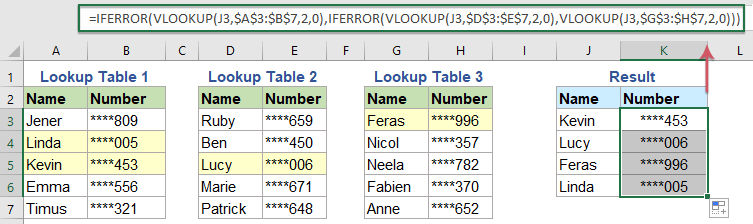Notes:

• 1. In the above formula, J3 is the value you are looking for; A3:B7, D3:E7, G3:H7 are the table ranges in which the lookup value and return value exist; The number 2 is the column number in the range to return the matching value from.
• 2. If the look up value can’t be found, an error value is displayed, to replace the error with a readable text, please use this formula: =IFERROR(VLOOKUP(J3,\$A\$3:\$B\$7,2,0),IFERROR(VLOOKUP(J3,\$D\$3:\$E\$7,2,0),IFERROR(VLOOKUP(J3,\$G\$3:\$H\$7,2,0),"can't find")))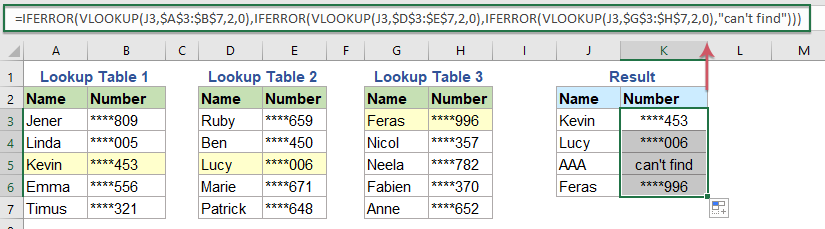#### 6. Vlookup to check if value exists based on a list data in another column

The Vlookup function also can help you to check if values exist based on another list, for example, if you want to look for the names in column C and just return Yes or No if the name is found or not in column A as below screenshot shown.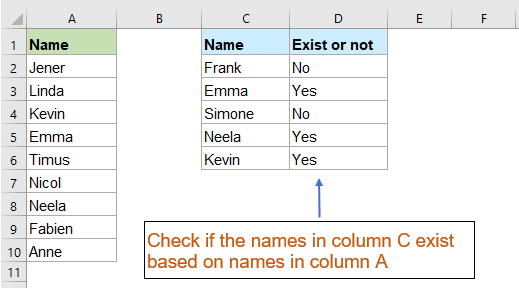1. Please apply the following formula into a blank cell:

=IF(ISNA(VLOOKUP(C2,\$A\$2:\$A\$10,1,FALSE)), "No", "Yes")

2. Then, drag the fill handle down to the cells that you want to fill this formula, and you will get the result as you need, see screenshot: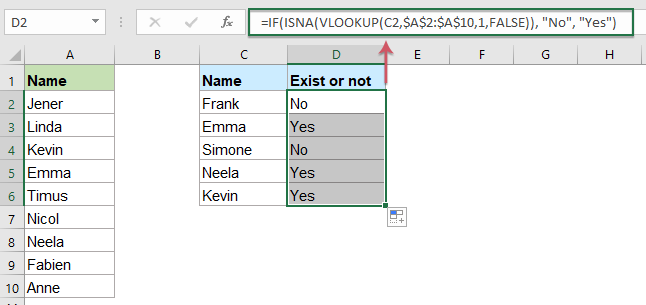Note: In the above formula, C2 is the lookup value you want to check; A2:A10 is the list of range from where lookup values will be found; the number 1 is the column number from where you want to fetch value in your range.

#### 7. Vlookup and sum all matched values in rows or columns

If you work with numerical data, sometimes, when extracting the matched values from the table, you may also need to sum the numbers in several columns or rows. This section will introduce some formulas to finish this job in Excel.

###### Vlookup and sum all matched values in a row or multiple rows

Suppose, you have a product list with sales for several months as below screenshot shown, now, you need to sum all orders in all months based on the given products.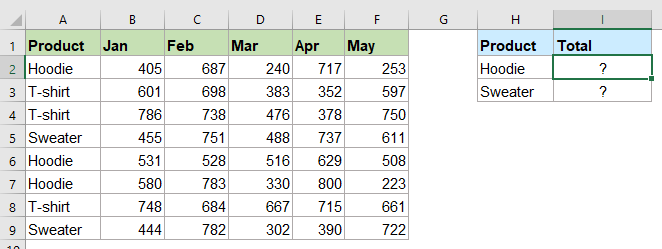Vlookup and sum the first matched values in a row:

1. Please copy or enter the following formula into a blank cell, and then press Ctrl + Shift + Enter keys together to get the first result.

=SUM(VLOOKUP(H2, \$A\$2:\$F\$9, {2,3,4,5,6}, FALSE))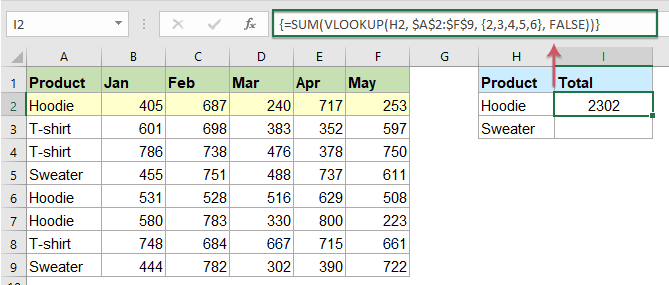2. Then, drag the fill handle down to copy this formula to other cells you need, and all the values in a row of the first matching value have been summed together, see screenshot: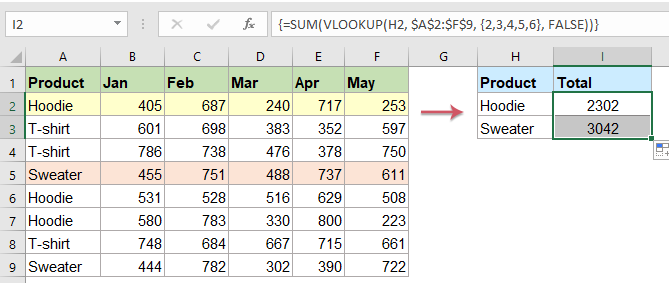Note: In the above formula: H2 is the cell containing the value you are looking for; A2:F9 is the data range (without column headers) which include the lookup value and the matched values; The number {2,3,4,5,6} are column numbers used to calculate the total of the range.

Vlookup and sum all matched values in multiple rows:

The above formula can only sum values in a row for the first matched value. If you want to sum all matches in multiple rows, please use the following formula, and then drag the fill handle down to the cells you want to apply this formula, and you will get the desired result you need, see screenshot:

=SUMPRODUCT((\$A\$2:\$A\$9=H2)*\$B\$2:\$F\$9)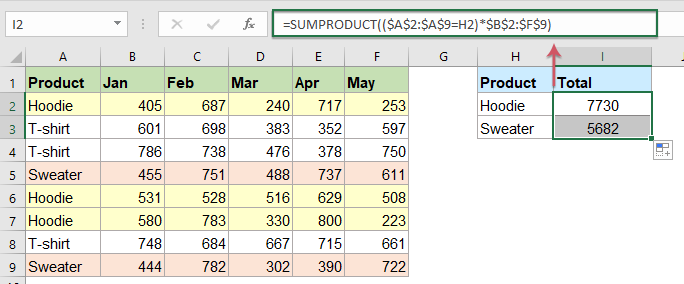Note: In the above formula: H2 is the lookup value you are looking for; A2:A9 is the row headers that contain the lookup value; B2:F9 the data range of the numeric values that you want to sum.

###### Vlookup and sum all matched values in a column or multiple columns

Vlookup and sum the first matched values in a column:

If you want to sum the total value for the specific months as shown in the screenshot below.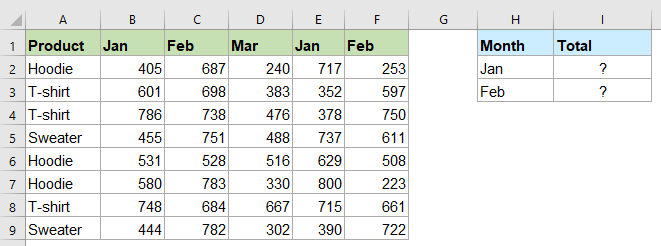Apply the below formula into a blank cell, and then drag the fill handle down to copy this formula to other cells, now, the first matched values based on the specific month in a column have been summed together, see screenshot:

=SUM(INDEX(\$B\$2:\$F\$9,0,MATCH(H2,\$B\$1:\$F\$1,0)))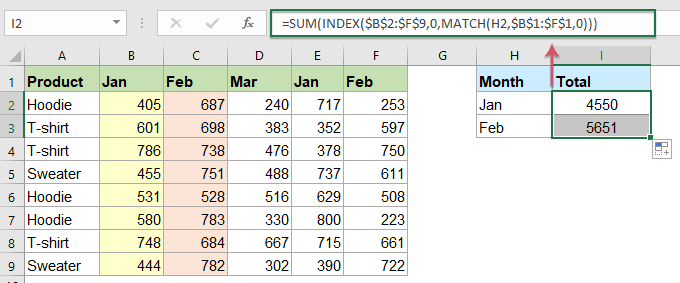Note: In the above formula: H2 is the lookup value you are looking for; B1:F1 is the column headers that contain the lookup value; B2:F9 the data range of the numeric values that you want to sum.

Vlookup and sum all matched values in multiple columns:

To Vlookup and sum all matched values in multiple columns, you should use the following formula:

=SUMPRODUCT(\$B\$2:\$F\$9*((\$B\$1:\$F\$1)=H2))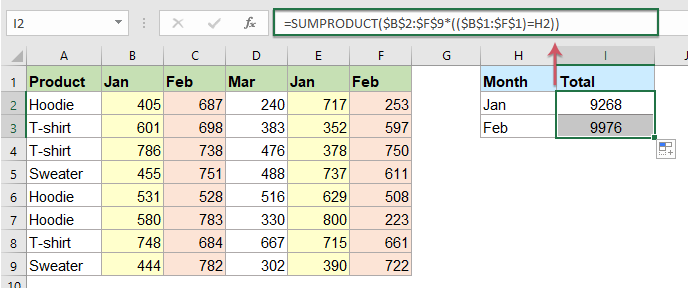Note: In the above formula: H2 is the lookup value you are looking for; B1:F1 is the column headers that contain the lookup value; B2:F9 the data range of the numeric values that you want to sum.

###### Vlookup and sum the first matched or all matched values with a powerful feature

Maybe the above formulas are difficult for you to remember, in this case, I will recommend a handy feature - Lookup and Sum of Kutools for Excel, with this feature, you can get the result as easy as possible.    Click to download Kutools for Excel now!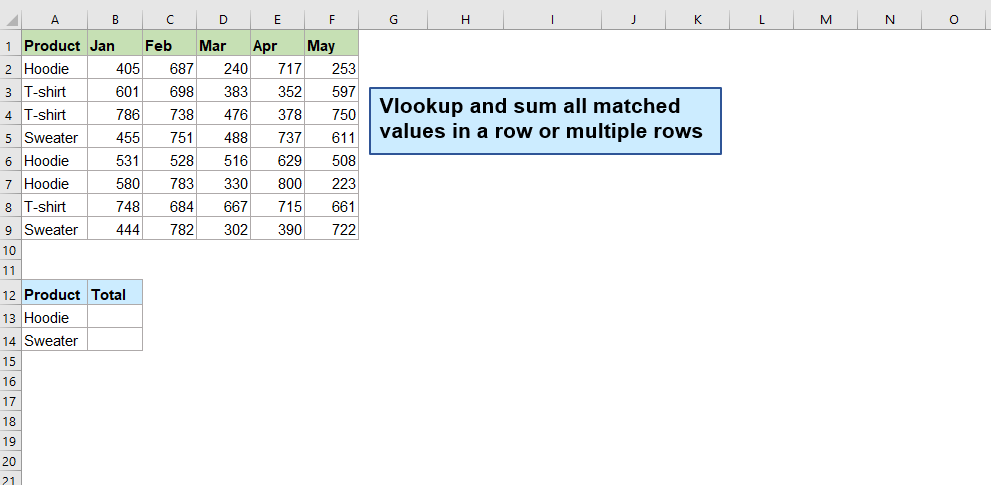###### Vlookup and sum all matched values both in rows and columns

If you want to sum the values when you need to match both column and row, for example, to get the total value of the product Sweater in month Mar as below screenshot shown.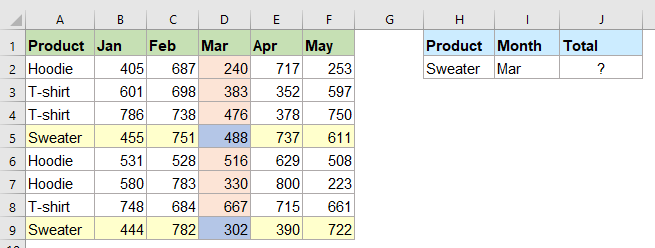Please apply the following formula into a cell, and then press Enter key to get the result, see screenshot:

=SUMPRODUCT((\$B\$2:\$F\$9)*(\$B\$1:\$F\$1=I2)*(\$A\$2:\$A\$9=H2))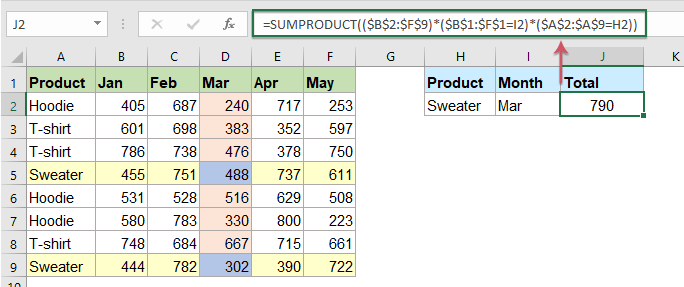Note: In the above formula: B2:F9 is the data range of the numeric values that you want to sum; B1:F1 is the column headers contain the lookup value that you want to sum based on; I2 is the lookup value within the column headers you are looking for; A2:A9 is the row headers contain the lookup value that you want to sum based on; H2 is the lookup value within the row headers you are looking for.

#### 8. Vlookup to merge two tables based on one or more key columns

In your daily work, when analyzing the data, you may need to gather all the necessary information into a single table based on one or more key columns. To solve this job, the Vlookup function also can do you a favor.

###### Vlookup to merge two tables based on one key column

For example, you have two tables, the first table contains the products and names data, and the second table contains the products and orders, now, you want to combine these two tables by matching the common product column into one table.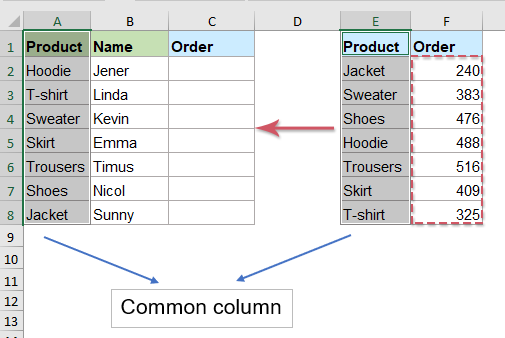Formula 1: Using VLOOKUP function

To merge the two tables into one based on a key column, please apply the following formula into a blank cell where you want to get the result, and then drag the fill handle down to the cells that you want to apply this formula, you will get a merged table with the order column joining to the first table data based on the key column data.

=VLOOKUP(\$A2,\$E\$2:\$F\$8,2,FALSE)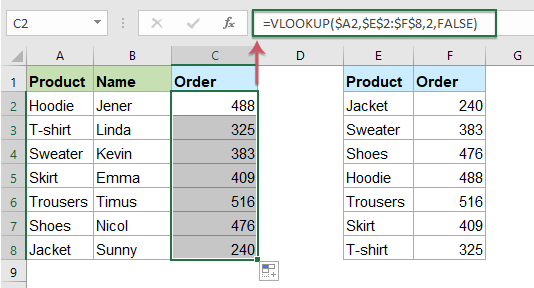Note: In the above formula, A2 is the value you are looking for, E2:F8 is the table to search, the number 2 is the column number in the table from which to retrieve the value.

Formula 2: Using INDEX and MATCH functions

If your common data in the right side and the returned data in the left column within the second table, to merge the order column, the Vlookup function is unable to do the job. To look up from right to left, you can use the INDEX and MATCH functions to replace the Vlookup function.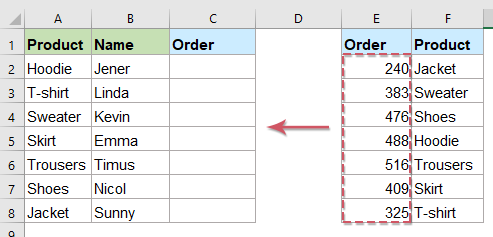Please copy or enter the below formula into a blank cell, then copy the formula down the column, and the order column has been joined to the first table, see screenshot:

=INDEX(\$E\$2:\$E\$8, MATCH(\$A2, \$F\$2:\$F\$8, 0))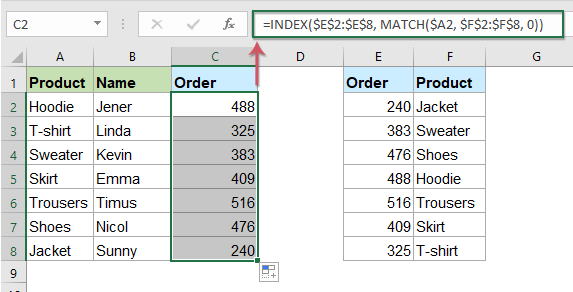Note: In the above formula, A2 is the lookup value you are looking for, E2:E8 is range of data that you want to return, F2:F8 is lookup range which contains the lookup value.

###### Vlookup to merge two tables based on multiple key columns

If the two tables you want to join have multiple key columns, to merge the tables based on these common columns, the INDEX and MATCH functions can help you.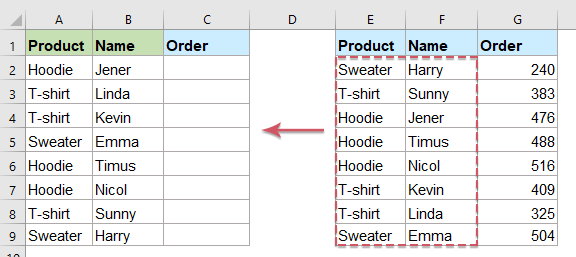The generic formula for merging two tables based on multiple key columns is:

=INDEX(lookup_table, MATCH(1, (lookup_value1=lookup_range1) * (lookup_value2=lookup_range2), 0), return_column_number)

1. Please apply the below formula into a blank cell where you want to put the result, and then press Ctrl + Shift + Enter keys together to get the first matched value, see screenshot:

=INDEX(\$E\$2:\$G\$9, MATCH(1, (\$A2=\$E\$2:\$E\$9) * (\$B2=\$F\$2:\$F\$9), 0), 3)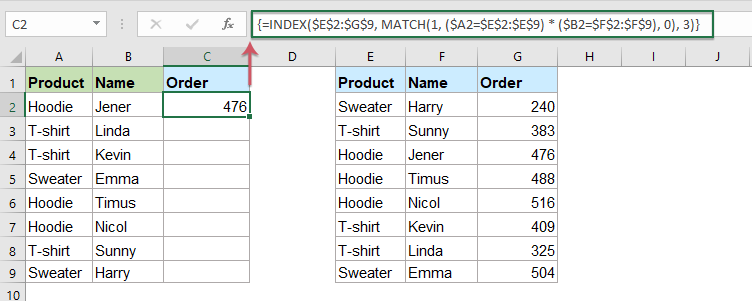Note: In the above formula, what the cell references represent as below screenshot shown: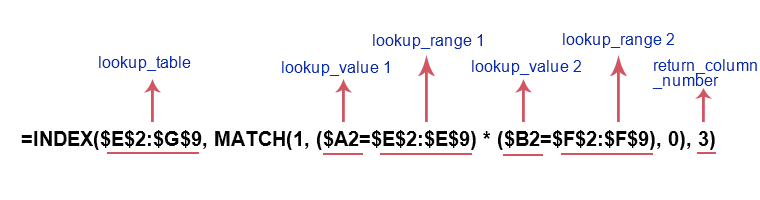2.Then, select the first formula cell, and drag the fill handle to copy this formula to other cells as you need: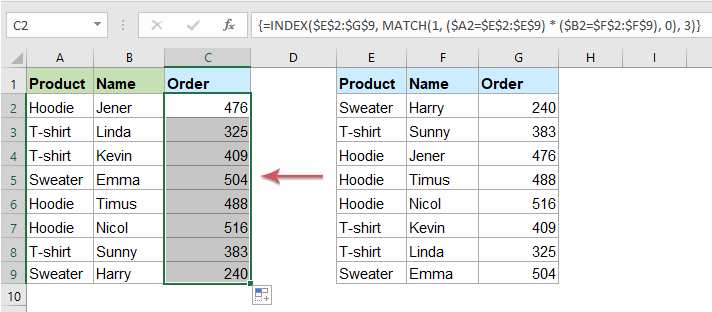Tips: In Excel 2016 and later versions, you can also use the Power Query feature to merge two or more tables into one based on key columns. Please click to know the details step by step.

#### 9. Vlookup matching values across multiple worksheets

Have you ever tried to Vlookup values across multiple worksheet? Supposing I have the following three worksheets with range of data, and now, I want to get part of the corresponding values based on the criteria from these three worksheets to get the result as below screenshot shown. In this case, the Vlookup Values Across Multiple Worksheets tutorial may do you a favor step by step.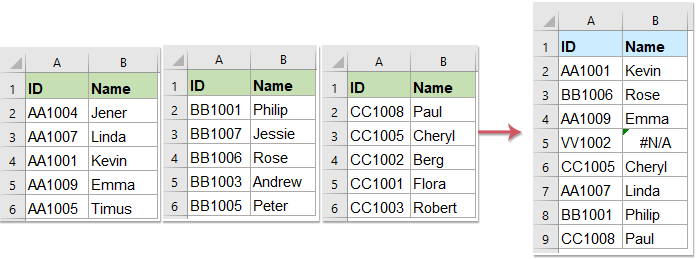### VLOOKUP matched values keep cell formatting

#### 1. Vlookup to get cell formatting (cell color, font color) along with lookup value

As we all know, the normal Vlookup function only can help us to return the matched value from another data range, but sometimes, you may want return the corresponding value along with the cell formatting, such as the fill color, font color, font style as below screenshot shown. This section will talk about how to get the cell formatting with the returned value in Excel.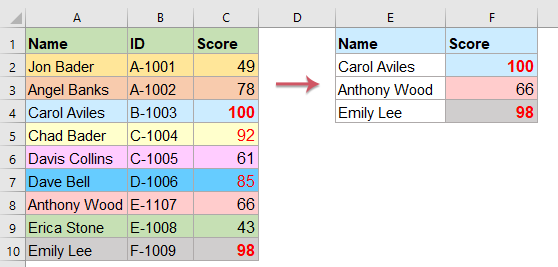Please do with the following steps to lookup and return its corresponding value along with cell formatting:

1. In the worksheet contains the data you want to Vlookup, right click the sheet tab and select View Code from the context menu. See screenshot: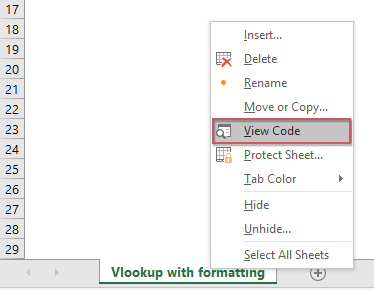2. In the opened Microsoft Visual Basic for Applications window, please copy below VBA code into the Code window.

VBA code 1: Vlookup to get cell formatting along with lookup value

``````Sub Worksheet_Change(ByVal Target As Range)
'Updateby Extendoffice
Dim I As Long
Dim xKeys As Long
Dim xDicStr As String
On Error Resume Next
Application.ScreenUpdating = False
xKeys = UBound(xDic.Keys)
If xKeys >= 0 Then
For I = 0 To UBound(xDic.Keys)
xDicStr = xDic.Items(I)
If xDicStr <> "" Then
Range(xDic.Keys(I)).Interior.Color = _
Range(xDic.Items(I)).Interior.Color
Range(xDic.Keys(I)).Font.FontStyle = _
Range(xDic.Items(I)).Font.FontStyle
Range(xDic.Keys(I)).Font.Size = _
Range(xDic.Items(I)).Font.Size
Range(xDic.Keys(I)).Font.Color = _
Range(xDic.Items(I)).Font.Color
Range(xDic.Keys(I)).Font.Name = _
Range(xDic.Items(I)).Font.Name
Range(xDic.Keys(I)).Font.Underline = _
Range(xDic.Items(I)).Font.Underline
Else
Range(xDic.Keys(I)).Interior.Color = xlNone
End If
Next
Set xDic = Nothing
End If
Application.ScreenUpdating = True
End Sub
``````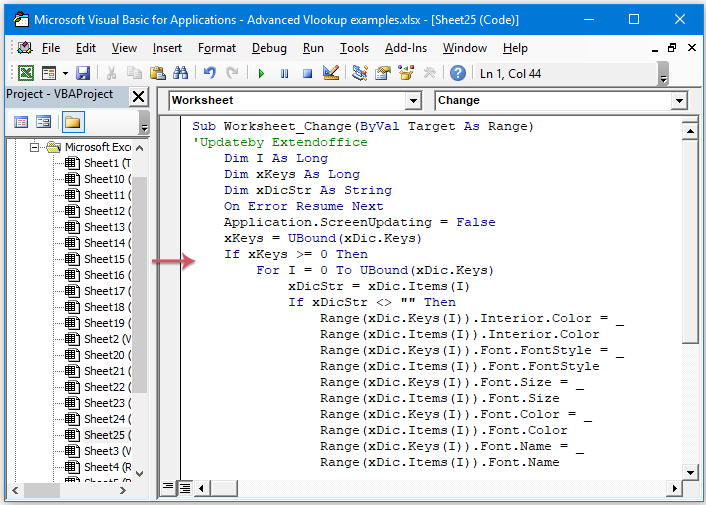3. Still in the Microsoft Visual Basic for Applications window, click Insert > Module, and then copy the below VBA code 2 into the Module window.

VBA code 2: Vlookup to get cell formatting along with lookup value

``````Public xDic As New Dictionary
Function LookupKeepFormat (ByRef FndValue, ByRef LookupRng As Range, ByRef xCol As Long)
Dim xFindCell As Range
On Error Resume Next
Set xFindCell = LookupRng.Find(FndValue, , xlValues, xlWhole)
If xFindCell Is Nothing Then
LookupKeepFormat = ""
Else
LookupKeepFormat = xFindCell.Offset(0, xCol - 1).Value
End If
End Function
``````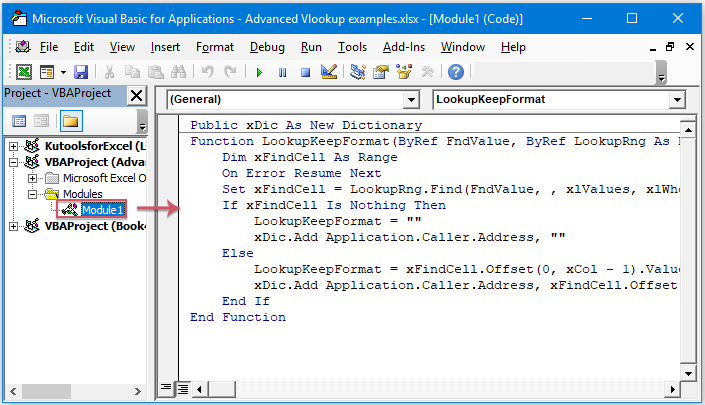4. After inserting the above codes, then click Tools > References in the Microsoft Visual Basic for Applications window. Then check the Microsoft Script Runtime checkbox in the References – VBAProject dialog box. See screenshots: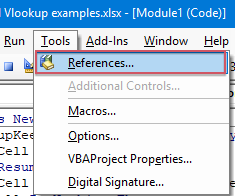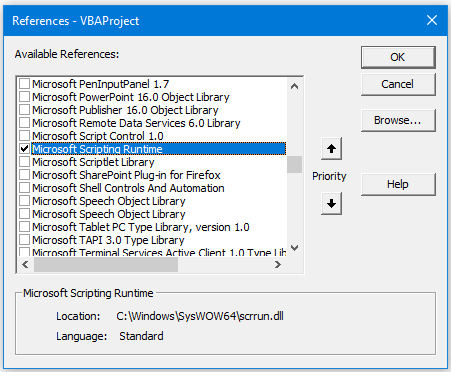5. Then, click OK to close the dialog box, and then save and close the code window, now, go back the worksheet, and then apply this formula: =LookupKeepFormat(E2,\$A\$1:\$C\$10,3) into a blank cell where you want to output the result, and then press the Enter key. See screenshot: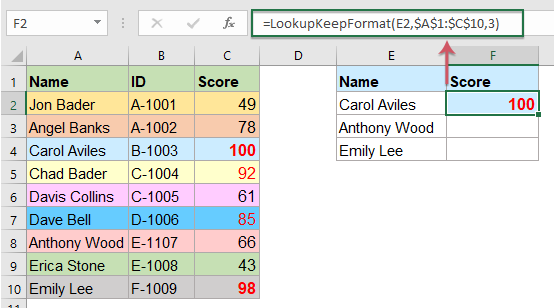Note: In the above formula, E2 is the value you will look up, A1:C10 is the table range, and number 3 is the column number of the table you want the matched value returned.

6. Then, select the first result cell, and drag the fill handle down to get all results along with their formatting. See screenshot.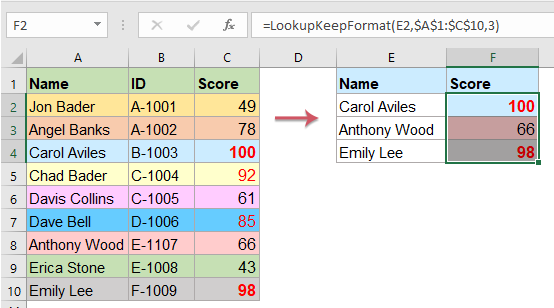#### 2. Keep the date format from a Vlookup returned value

Normally, when using the Vloook function to look up and return the matched date format value, some number format will be displayed as below screenshot shown. To keep the date format from a returned result, you should enclose the TEXT function to the Vlookup function.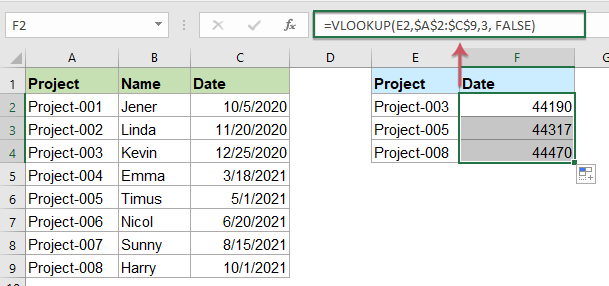Please apply the below formula into a blank cell, and then drag the fill handle to copy this formula to other cells, and all the matched dates have been returned as below screenshot shown:

=TEXT(VLOOKUP(E2,\$A\$2:\$C\$9,3,FALSE),"mm/dd/yyyy")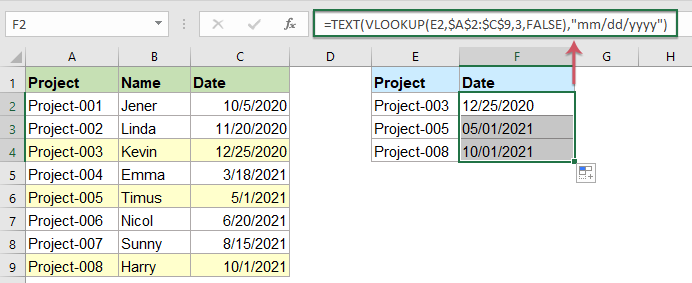Note: In the above formula, E2 is the look value, A2:C9 is the lookup range, the number 3 is the column number you want the value returned, mm/dd/yyyy is the date format you want to keep.

#### 3. Vlookup and return matching value with cell comment

Have you ever tried to Vlookup to return not only the matched cell data, but also the cell comment as well in Excel as following screenshot shown? To solve this task, the below User Defined Function can do you a favor.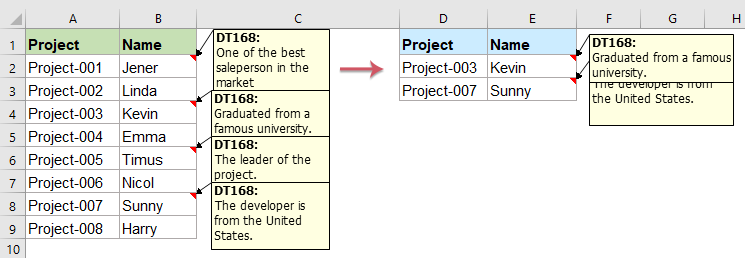1. Hold down the ALT + F11 keys to open the Microsoft Visual Basic for Applications window.

2. Click Insert > Module, then copy and paste the following code in the Module Window.

VBA code: Vlookup and return matching value with cell comment:

```Function VlookupComment(LookVal As Variant, FTable As Range, FColumn As Long, FType As Long) As Variant
'Updateby Extendoffice
Application.Volatile
Dim xRet As Variant 'could be an error
Dim xCell As Range
xRet = Application.Match(LookVal, FTable.Columns(1), FType)
If IsError(xRet) Then
Else
Set xCell = FTable.Columns(FColumn).Cells(1)(xRet)
VlookupComment = xCell.Value
With Application.Caller
If Not .Comment Is Nothing Then
.Comment.Delete
End If
If Not xCell.Comment Is Nothing Then
End If
End With
End If
End Function
```

3. Then save and close the code window, enter this formula: =vlookupcomment(D2,\$A\$2:\$B\$9,2,FALSE) into a blank cell to locate the result, and then drag the fill handle to copy this formula to other cells, now, the matched values as well as the comments are returned at once, see screenshot: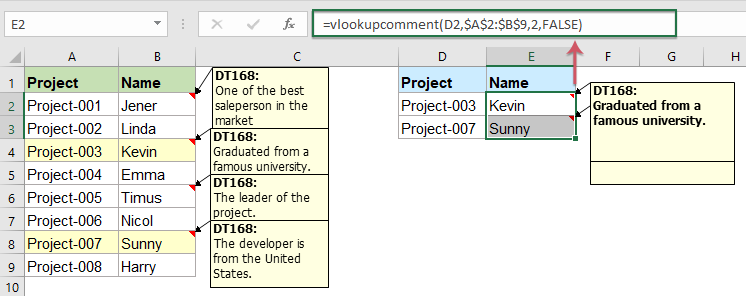Note: In the above formula, D2 is the lookup value you want to return its corresponding value, A2:B9 is the data table you want to use, the number 2 is the column number which contains the matched value you want to return.

#### 4. Deal with the text and real numbers in Vlookup

For instance, I have a range of data, the ID number in the original table is number format, in the lookup cell which is stored as text, when applying the normal Vlookup function, an #N/A error result is displayed as below screenshot shown. In this case, how could you get the correct information if the lookup number and original number in table have the different data format?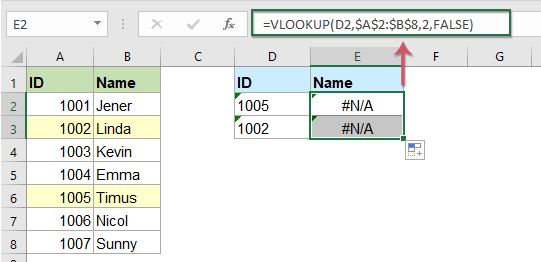For dealing with the text and real numbers in Vlookup function, please apply the following formula into a blank cell, and then drag the fill handle down to copy this formula, and you will get the correct results as below screenshot shown:

=IFERROR(VLOOKUP(VALUE(D2),\$A\$2:\$B\$8,2,0),VLOOKUP(TEXT(D2,0),\$A\$2:\$B\$8,2,0))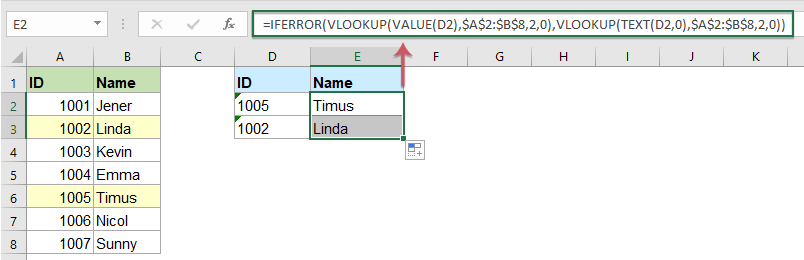Notes:

• 1. In the above formula, D2 is the lookup value you want to return its corresponding value, A2:B8 is the data table you want to use, the number 2 is the column number which contains the matched value you want to return.
• 2. This formula also works well if you are not sure where you have numbers and where you have text.

Vlookup_basic_examples.xlsx

Vlookup_keep_cell_formatting.zip

## The Best Office Productivity Tools

### Kutools for Excel Solves Most of Your Problems, and Increases Your Productivity by 80%

• Super Formula Bar (easily edit multiple lines of text and formula); Reading Layout (easily read and edit large numbers of cells); Paste to Filtered Range...
• Merge Cells/Rows/Columns and Keeping Data; Split Cells Content; Combine Duplicate Rows and Sum/Average... Prevent Duplicate Cells; Compare Ranges...
• Select Duplicate or Unique Rows; Select Blank Rows (all cells are empty); Super Find and Fuzzy Find in Many Workbooks; Random Select...
• Exact Copy Multiple Cells without changing formula reference; Auto Create References to Multiple Sheets; Insert Bullets, Check Boxes and more...
• Favorite and Quickly Insert Formulas, Ranges, Charts and Pictures; Encrypt Cells with password; Create Mailing List and send emails...
• Extract Text, Add Text, Remove by Position, Remove Space; Create and Print Paging Subtotals; Convert Between Cells Content and Comments...
• Super Filter (save and apply filter schemes to other sheets); Advanced Sort by month/week/day, frequency and more; Special Filter by bold, italic...
• Combine Workbooks and WorkSheets; Merge Tables based on key columns; Split Data into Multiple Sheets; Batch Convert xls, xlsx and PDF...
• Pivot Table Grouping by week number, day of week and more... Show Unlocked, Locked Cells by different colors; Highlight Cells That Have Formula/Name...### Office Tab - brings tabbed interface to Office, and make your work much easier

• Enable tabbed editing and reading in Word, Excel, PowerPoint, Publisher, Access, Visio and Project.
• Open and create multiple documents in new tabs of the same window, rather than in new windows.
• Increases your productivity by 50%, and reduces hundreds of mouse clicks for you every day!